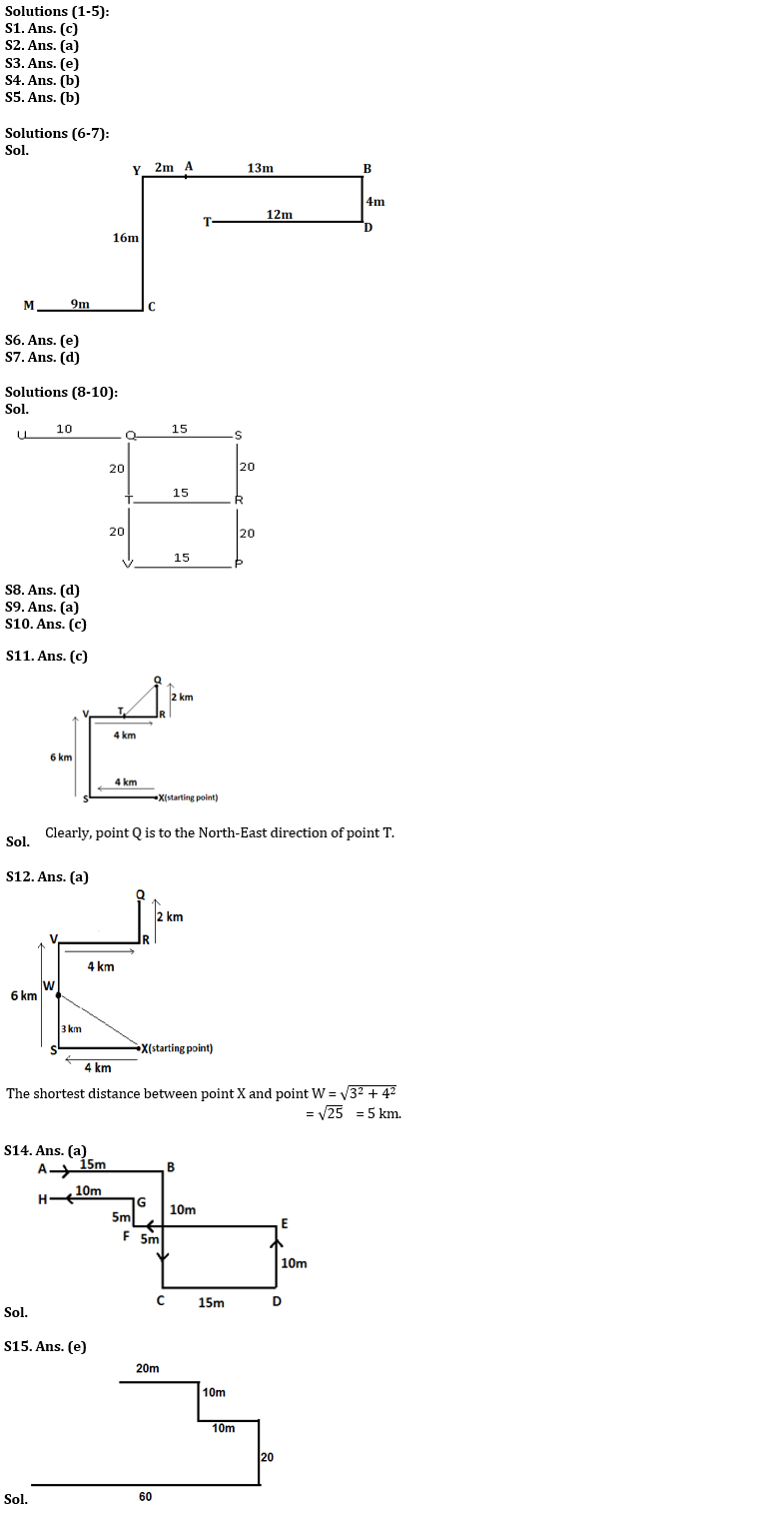Latest Banking jobs   »   Reasoning Ability Quiz For SBI PO...

# Reasoning Ability Quiz For SBI PO Prelims 2022- 28th November

Directions (1-5): Study the following alphabetical sequence and answer the questions following it.

A B B C D E F E I B C A F E C B B A C A O B N U V W

Q1. How many B’s are there in the alphabetical series which are immediately preceded by a vowel and immediately followed by consonant?
(a) One
(b) Two
(c) Three
(d) More than three
(e) None of these

Q2. If all the vowels are dropped from the series, then which alphabet will be eighth from the right end?
(a)C
(b)B
(c) N
(d)F
(e) None of these

Q3. How many consonants are there in the alphabetical series which are immediately preceded by a Vowel?
(a) One
(b) Two
(c) Four
(d) None
(e) More than Five

Q4. If the position of the 1st and the 14th alphabets, the 2nd and the 15th alphabets, and so on up to the 13th and the 26th alphabets, are interchanged, then which alphabet will be 7th to the right of 10th alphabet from the right end?
(a) A
(b) C
(c) N
(d)B
(e) None of these

Q5. How many total vowels are there in the alphabetical series?
(a) Five
(b)Ten
(c)Three
(d) Nine
(e) None of these

Direction (6-7): Study the given information and answer the following question.
Point A is 13m west of point B. Point D is 4m south of point B. Point C is east of 9m of point M. Point T is 12m west of point D. Point Y is 16m north of point C. Point Y is 2m west of point A.

Q6. What is the direction of C with respect of T?
(a) North-west
(b) South
(c) North-east
(d) West
(e) None of these

Q7. In which direction of Point A with respect to Point T?
(a) North
(b) North-east
(c) East
(d) North-west
(e) None of these

Directions (8-10): Study the following information carefully and answer the given questions.
Point S is 40 m towards the North of Point P. Point Q is 15 m towards the West of point S. Point U is 25 m towards the West of point S. Point T is 20 m towards the South of point Q. Point R is 15 m towards the East of point T. Point V is 15 m towards the West of point P.

Q8. Which of the following points are in a straight line?
(a) S, T, P
(b) T, V, R
(c) S, Q, V
(d) T, V, Q
(e) U, Q, R

Q9. P is in which direction with respect to R?
(a) South
(b) North
(c) East
(d) West
(e) Cannot be determined

Q10. If a person walks 10 m towards East from point U and then takes a right turn, which of the following points would he reach first?
(a) V
(b) S
(c) T
(d) P
(e) R

Directions (11-12): Read the following information carefully and answer the given questions.
A man starts waking from a point X to point S in the west direction and covers 4 km. He then turned to his right and walks for 6 km to reach at a point V, from here he again took a right turn and walks for 4 km to reach at a point R. He finally turned to his left and walks for another 2 km to reach at a final point Q.

Q11. If point T is in the midway of point V and point R, then in which direction point Q is with respect to point T?
(a) North
(b) South West
(c) North East
(d) North West
(e) None of these

Q12. If point W is just in the middle of point S and point V, then what is the shortest distance between point X and point W?
(a) 5 km
(b) 7 km
(c) 6 km
(d) 4 km
(e) None of these

Directions (13-14): Study the information carefully and answer the questions given below.
A person starts walking from A to reach B, which is 15m east to A. He then takes a right turn and walks 20 m to reach point C. From C, he takes left turn and walks15m to point D, then again he takes a left turn and walks 10m to point E. From E, he takes a left turn and walks 20m to reach point F. He then takes a right turn and walks 5m to reach G and finally takes a left turn to reach point H, which is 10 m away from G.

Q13. If J is 5m north to G, then what is the distance between A and J?
(a) 5m
(b) 10m
(c) 15m
(d) 20m
(e) none of these

Q14. How far is point A from point H?
(a) 5m
(b) 10m
(c) 15m
(d) 20m
(e) none of these

Q15. Swati walks 20 m towards east. Then She takes right again and walk 10 m. Then she turns left and walks 10 m and then turning right and walk 20 m. Then she turns right again and walk 60 m. She is in which direction from her starting point?
(a) North
(b) North-west
(c) East
(d) North-east
(e) South-west

Solutions#### Congratulations!Union Budget 2023-24: Free PDF